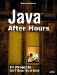# Drawing Some Graphics

The central method in Painter is the paint method. This is where all the drawing operations take placedrawing lines, ellipses, rectangles, text, with a wide variety of effects. To make those effects happen, Painter uses Java 2Dthat is, Graphics2D objects, not the Graphics objects used in the previous chapters.

To let the user expand and reduce figures interactively while dragging the mouse, Painter uses two different Graphics2D objectsone corresponding to the internal image where the final figure is stored when the user is done drawing, and one corresponding to the window while the user is dragging the mouse. To make the drawing operations easier, the current graphics context is stored in a variable named gImage, and all drawing operations then can simply use that object. Here's how gImage is set in the paint method, depending on whether or not the user is dragging the mouse. Note that the code backs up the Composite object, used in drawing operations, from gImage in case that object is changed later and needs to be reset (more on the Composite object in a few pages). Also, note that the code restricts drawing operations to the drawing area with the clip method. Here's the code:

` public void paint (Graphics g) {     Graphics2D gImage;     if(!dragging && !adjusted){         if(image == null){             image = createImage(imageWidth, imageHeight);         }         gImage = (Graphics2D) image.getGraphics();     }     else{         gImage = (Graphics2D) g;         g.drawImage(image, offsetX, offsetY, this);         g.drawRect(offsetX, offsetY, imageWidth, imageHeight);         gImage.clip(new Rectangle2D.Double(offsetX, offsetY,             imageWidth, imageHeight));     }         composite = gImage.getComposite();     .     .     . `

You can see the significant methods of the Graphics2D class in Table 4.2. That's the class Painter uses to draw and produce graphics effects, and it's worthwhile getting to know what's available here.

##### Table 4.2. The Significant Methods of the java.awt.Graphics2D Class

Method

Does This

abstract void draw(Shape s)

Draws a shape using the current settings in the Graphics2D context

` void draw3DRect(int x, int y, int width, int height, boolean raised) `

Draws a 3D outline of the given rectangle

` abstract  void drawImage(BufferedImage img, BufferedImageOp op, int x, int y) `

Draws an image that is first filtered with theBufferedImageOp object (which can be null)

` abstract  void drawString(String str, int x, int y) `

Draws the text of the given String object, using the current text drawing settings in the Graphics2D context

abstract void fill(Shape s)

Fills in the interior of a shape using the current settings of the Graphics2D context

` void fill3DRect(int x, int y, int width, int height, boolean raised) `

Fills in a 3D highlighted rectangle with thecurrent color

abstract Color getBackground()

Returns the current background color (used when clearing an area)

abstract Composite getComposite()

Returns the current Composite object used in the Graphics2D context

abstract Paint getPaint()

Returns the current Paint object used in the Graphics2D context

abstract void setBackground(Color color)

Sets the background color used in this Graphics2D context

abstract void setComposite(Composite comp)

Sets the Composite object used in the Graphics2D context

abstract void setPaint(Paint paint)

Sets the Paint attribute used in the Graphics2D context

Now that the drawing graphics context is set in the gImage object, you can configure that object according to the graphics effects the user has selected. If the user has selected a drawing color (more on how that works, using a color chooser dialog box, later), you can install that color as the drawing color in the graphics context, like this:

` if(color != null){     gImage.setColor(color); } else {     gImage.setColor(new Color(0, 0, 0)); } . . . `

Also, if the user has selected the Effects menu's Draw thick lines menu item, you can use the Graphics2D object's setStroke method to set the width of lines to a thicker value (Painter uses 10 pixels):

` if(thick){     gImage.setStroke(new BasicStroke(10)); } else{     gImage.setStroke(new BasicStroke(1)); } . . . `

Now the code can start drawing operations. If the mouse goes up, or the user is dragging the mouse, Painter is supposed to be drawing, so it checks the mouseUp and dragging flags.

Because several of the Graphics2D drawing methods require you to pass the upper-left point of the figure to draw, the code has to order the start and end points to determine the upper-left point (which can change as the user drags the mouse). Painter does this by creating two new points, tempStart and tempEnd, that are ordered as the Graphics2D drawing methods want, and it also finds the height and width of the figure:

` if (mouseUp || dragging) {     Point tempStart, tempEnd;     tempStart = new Point(Math.min(end.x, start.x),         Math.min(end.y, start.y));     tempEnd = new Point(Math.max(end.x, start.x),         Math.max(end.y, start.y));     tempStart = new Point(Math.max(tempStart.x, offsetX),         Math.max(tempStart.y, offsetY));     tempEnd = new Point(Math.max(tempEnd.x, offsetX),         Math.max(tempEnd.y, offsetY));     tempStart = new Point(Math.min(tempStart.x,         bufferedImage.getWidth() + offsetX),         Math.min(tempStart.y, bufferedImage.getHeight() +         offsetY));     tempEnd = new Point(Math.min(tempEnd.x,         bufferedImage.getWidth() + offsetX),         Math.min(tempEnd.y, bufferedImage.getHeight() +         offsetY));     if(!adjusted){         tempEnd.x -= offsetX;         tempEnd.y -= offsetY;         tempStart.x -= offsetX;         tempStart.y -= offsetY;         end.x -= offsetX;         end.y -= offsetY;         start.x -= offsetX;         start.y -= offsetY;         adjusted=true;     }     int width = tempEnd.x - tempStart.x;     int height = tempEnd.y - tempStart.y; } . . . `

That gets the setup out of the way. Now you're ready to draw using the graphics context in gImage. The first type of figure Painter takes care of is lines.Java After Hours: 10 Projects Youll Never Do at Work
ISBN: 0672327473
EAN: 2147483647
Year: 2006
Pages: 128

Similar book on Amazon